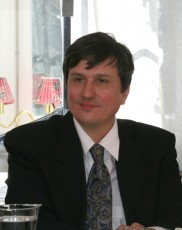GFM

Sections
You are here: Home Towards non-commutative integrability

Towards non-commutative integrability

Colloquium
Instituto para a Investigação Interdisciplinar, Anfiteatro
2010-07-07 14:30 .. 15:30
Contact: Jean-Claude Zambrini

A Pedro Nunes Lecture by Maxim Kontsevich (Institut des Hautes Études Scientifiques)

There are many examples of classical integrable systems where one can treat coordinates on the phase space as free non-commuting variables.

I'll mostly concentrate on an example related with the classification of a pair of matrices up to conjugation.

This leads to a beautiful class of (non)commutative algebraic functions.

Also I formulate several conjectures about integrability, supported by computer experiments.web streaming: http://www.sp.ul.pt/maximkontsevich

Professor Maxim Kontsevich's work concentrates on geometric aspects of mathematical physics, most notably on knot theory, quantization, and mirror symmetry. His most famous result is a formal deformation quantization that holds for any Poisson manifold. He also introduced knot invariants defined by complicated integrals analogous to Feynman integrals. In topological field theory, he introduced the moduli space of stable maps, which may be considered a mathematically rigorous formulation of the Feynman integral for topological string theory.

He received a Fields Medal in 1998, at the 23rd International Congress of Mathematicians in Berlin. He also received the Henri Poincaré Prize in 1997 and a Crafoord Prize in 2008.

« June 2019 »
Su Mo Tu We Th Fr Sa
1
2345678
9101112131415
16171819 202122
23242526 272829
30
Past conference highlightsSymmetries in Quantum Physics 2017Advances in Mathematical Fluid Mechanics, Stochastic & Deterministic MethodsQuantum Integrable Systems and GeometryChern-Simons Gauge Theory: 20 years afterSecond Workshop on Quantum Gravity and Noncommutative GeometryInfinite Dimensional Algebras and Quantum Integrable Systems IIStochastic Analysis in Mathematical PhysicsMathematics in ChemistryGeometric Aspects of Integrable SystemsICMP 2003